# Super-group

(diff) ← Older revision | Latest revision (diff) | Newer revision → (diff)

Lie super-group

A group object in the category of super-manifolds (cf. Super-manifold). A super-group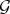is defined by a functorfrom the category of commutative superalgebras into the category of groups. Lie's theorems (cf. Lie theorem) are transferred to super-groups, a fact that gives the correspondence between super-groups and finite-dimensional Lie superalgebras (cf. Superalgebra).

### Examples.

1) The super-group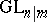is defined by the functor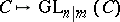into groups of even invertible matrices from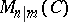(see Super-space), i.e. of matrices in the formwhere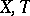are invertible matrices of orders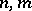over, while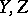are matrices over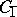. A homomorphismis defined by the formula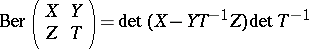(the Berezinian);

2)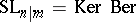;

3)and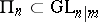; they leave invariant an even, or odd, non-degenerate symmetric bilinear form.

To every super-groupand super-subgroupof it there is related a super-manifold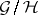, represented by a functor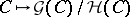. This super-manifold is a homogeneous space of.

How to Cite This Entry:
Super-group. Encyclopedia of Mathematics. URL: http://encyclopediaofmath.org/index.php?title=Super-group&oldid=17496
This article was adapted from an original article by D.A. Leites (originator), which appeared in Encyclopedia of Mathematics - ISBN 1402006098. See original article